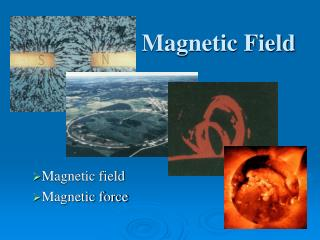DownloadDownload PresentationMagnetic Field

# Magnetic Field

Télécharger la présentation## Magnetic Field

- - - - - - - - - - - - - - - - - - - - - - - - - - - E N D - - - - - - - - - - - - - - - - - - - - - - - - - - -
##### Presentation Transcript

1. Magnetic Field Magnetic field Magnetic force

2. Magnetic Field Theories in Physics Classical Mechanics Thermodynamics Electromagnetic Relativity Quantum Mechanics

3. Theories in Physics Relativity Quantum Electrodynamics Classical Mechanics Electromagnetic Quantum Mechanics Electricity Magnetism Statistical Mechanics Optics Thermodynamics Magnetic Field

4. Theories in Physics Areas of Physics Relativity Quantum Electrodynamics Electromagnetic Electricity Magnetism Optics Magnetic Field

5. Reading Question The magnetic field of a point charge is given by 1. Biot-Savart’s law. 2. Faraday’s law. 3. Gauss’s law. 4. Ampère’s law. 5. Einstein’s law.

6. Reading Question The magnetic field of a point charge is given by 1. Biot-Savart’s law. 2. Faraday’s law. 3. Gauss’s law. 4. Ampère’s law. 5. Einstein’s law.

7. Reading Question The magnetic field of a straight, current-carrying wire is 1. parallel to the wire. 2. inside the wire. 3. perpendicular to the wire. 4. around the wire. 5. zero.

8. Reading Question The magnetic field of a straight, current-carrying wire is 1. parallel to the wire. 2. inside the wire. 3. perpendicular to the wire. 4. around the wire. 5. zero.

9. Student Workbook

10. Student Workbook What about this? A plastic ball has a positive charge and is brought near an uncharged plastic ball. What happens?

11. Student Workbook

12. Student Workbook

13. Magnetic Field • The first thing we would like to do is to compare the magnetic field to the electric field. What does the electric field lines for a positive point charge look like. • Next draw the magnetic field lines for the bar magnetic which is the same as a electric dipole. + N S

14. Magnetic Field The field is displayed by using Iron filings

15. Magnetic Field The field is displayed by using Iron filings

16. N S N S Magnetic Field • What would happen if you broke the bar magnetic in half? Discuss this in your group and come up with an answer. Draw the magnetic field lines for one-half of the broken magnet. • If you were to continue to break the bar magnet in half, would you ever get to where you had just a north pole or just a south pole? Discuss this in your group and write your answer.

17. Magnetic Field

18. Magnetic Field

19. Magnetic Field

20. Magnetic Field

21. Magnetic Field

22. Magnetic Field • Magnetic Field Due to a Current Element Let’s compare this to the electric field due to a charge element. What is the equation?

23. Magnetic Field

24. Magnetic Field A long straight current caring wire

25. Magnetic Field

26. Magnetic Field • Thus one difference is that • there are no magnetic monopoles, they always come in pairs, N and S, magnetic dipole • What does this tell us if we try to write a Gauss’ type law for magnetism? • Discuss this in your group and explain.

27. N S Magnetic Field • Draw the electric field lines for an electric dipole. • Compare the field lines for the magnetic dipole and the electric dipole. What do you note? + -

28. Student Workbook

29. Student Workbook